# Ecological Committee on FIFRA Risk Assessment Methods (ECOFRAM) Terrestrial Workgroup Report: V. Risk Characterization

## Introduction

Risk characterization is a final stage of ecological risk assessment where results of exposure and ecological effects analyses are integrated to evaluate the likelihood of adverse ecological effects occurring following exposure to a stressor. The ecological significance of the adverse effects should be discussed, including consideration of the types and magnitudes of the effects, their spatial and temporal patterns, and the likelihood of recovery (USEPA, 1992). This poster discusses methods for risk assessment for pesticides. Risk assessment is the analysis component of the risk characterization that integrates exposure and effects assessments to provide estimates of risk and evaluates uncertainties (USEPA, 1998). Risk estimates should be pertinent to the assessment endpoints that were defined in the Problem Formulation stage. The primary assessment endpoints determined by the ECOFRAM Terrestrial Workgroup were:

1. Effects on the survival and reproduction of individual birds and mammals.

2. Effects on population size and persistence of birds and mammals.

The risk characterization needs to place the output of the risk assessment in perspective and provide concise information that can be used for risk management. If the information is insufficient to support decisionmaking by risk managers, or the risk assessment needs to be further refined, it may be necessary to proceed to a further iteration of the risk assessment or to a higher level of refinement in the risk assessment process (see Levels of Refinement).

Top of page

## Overview of Risk Assessment Methods

A suite of risk assessment methods are recommended in order to provide the flexibility necessary to manage the diversity of pesticide scenarios for which a risk assessment is necessary (Fig. 1). Methods within the suite are grouped according to the level of sophistication, effort required, data required, and extent of refinement of the risk assessment. These methods can generally be divided into three categories:

1. Deterministic quotients

2. Comparison of exposure distribution to effects distribution (or fixed value)

3. Integration of exposure and effects distributions (Fig. 1; Methods 4, 5 and 6)

Top of page

## Examples of Risk Assessment Methods

Examples based on hypothetical data sets were developed to illustrate ecological risk assessment Methods 1 through 5. These examples are not case studies and do not provide a proof-of-concept but do allow a conceptual comparison of the methods and their risk assessment outputs. The examples use a single distribution of exposure values and three different sets of toxicity data, as follows:

##### The exposure data (mg/kg/d) to the left were fitted to a distribution below.

Distribution Type = Lognormal
Mean = 77.61
Standard Deviation = 40.05
95 percentile = 153.37
90 percentile = 128.56

Hypothetical Exposure Data
Exposure
mg/kg/d
%
Probability
%
Cumulative
301010
331020
451030
601040
811050
881060
891070
951080
1201090
12610100

Toxicity Data Sets

Set 1
LD50
mg/kg/d
%
Prob.
%
Cum.
902525
1202550
2502575
35025100
Set 3
LD50
mg/kg/d
95%tile
mg/kg/
5%tile
mg/kg/d
220260180
Set 2
LD50
mg/kg/d
%
Cum.
15020
15540
19560
21080
350100
The toxicity data (mg/kg/d) were fitted to a distribution as follows: Toxicity Data Set 1
ParameterValue
Distribution TypeLognormal
Mean203.51
Standard Deviation119.92
5 percentile71.43
10 percentile87.1

For each example, details of the output information on risk is provided to illustrate how the output could be interpreted. However, interpretation of the risk output is dependent on the exposure and effects inputs and what these represent. The explanation of risk is also dependent on the question being asked. The probabilistic risk assessment examples were generated using Crystal Ball, an EXCEL add-in for conducting model simulations. Using this software (and similar software e.g, @Risk) it is very easy to view data and to fit distributions to data. Distributions can be use to instead of a fixed value to represent the uncertainty around this value. Where actual data are available, these data should be preferentially used and the appropriate distribution should be carefully fitted to data. Selection of distributions based on minimal data, or data that poorly fit the distribution, should be used with caution.

Ratio of single values of exposure and effects Risk based on a comparison of exposure and effect distributions (Probability of Exceeding a Fixed Value) ↑ ↑ ↑ Less Realistic Risk Assessment ↑ ↑ ↑ Method 1 Point Estimate Quotients (deterministic) Exposure: Point estimate of exposure (mg/kg b.w./ unit time) Effects: Point estimate of toxicity (e.g., NOEL, LD50) Output: A ratio of exposure/toxicity (relative to risk but risk is not quantified) Method 2 Comparison of Exposure Distribution with Point Estimate for Effects Exposure: Distribution of exposure (mg/kg b.w./ unit time) Effects: Point estimate of toxicity (e.g., NOEL, LD50) Output: Probability of exposure exceeding the effect level Method 3 Comparison of Exposure and Effects Distributions (degree of overlap) Exposure: Cumulative frequency distribution of exposure (mg/kg b.w./ unit time) Effects: Distribution of toxicity for i. various species or ii. single species (e.g., LD50) Output: Probability of certain effect occurring when a fixed exposure level is exceeded ↓↓↓More Realistic Risk Assessment ↓↓↓ Method 4 Distribution-Based Quotients (uses Monte Carlo Simulations) Exposure: Distribution of exposure (mg/kg b.w./ unit time) Effects: Distribution of toxicity for i. various species or ii. single species (e.g., LD50) Output: Probability distribution of quotients (probability that exposure exceeds toxicity) Method 5 Integrated Exposure and Effects Distributions (uses Monte Carlo Simulations) Exposure: Distribution of exposure (mg/kg b.w./ unit time) Effects: Distribution of toxicity (dose response distribution) Output: Probability of certain magnitude of effect (mortality) occurring Method 6 Mechanistic/Process models Stage/Age structured; Meta-population; Individual-based; Spatially explicit models

Top of page

## Method 1: Point Estimate Quotients

In the FIFRA regulatory process to date, the quotient method has been used in risk assessment for pesticides. A quotient of single values for exposure and effects are calculated (exposure value/toxicity value) and if the quotient exceeds a trigger value (equal to or less than 1), an adverse effect is considered likely to occur. The quotient values do not quantify risk but provide results that are relative to risk. An example assessment using the point estimate quotients approach is as follows:

Inputs for Exposure and Effects
(Toxicity Data Set 1)
Exposure
mg/kg/d
Effects
mg/kg/d
Quotient Value
(Exposure/Effects)
Based on 95 and 5 %tile15371.42.14
Based on 90 and 10%tile128.687.11.48
Based on worst case data points126901.40

The exposure values exceed the LD50 values resulting in quotient values greater than 1. This assessment does not indicate that an effect is unlikely. It does indicate that a refined assessment is necessary to determine the risk. The assessment provides no information on

1. the probability of an effect occurring or
2. the size of the effect.

Top of page

## Method 2: Comparison of Exposure Distribution and Point Estimate for Effects

In terrestrial vertebrate risk assessment, data supporting exposure assessments are likely more readily available than toxicity data. Consequently, models and resulting distributions are more easily obtainable for characterizing exposure than toxic effects. In this method, a single distribution of exposure is generated and a point estimate of toxicity is selected. Risk is estimated based on the probability of the effect level occurring within the distribution of exposure. This method is applicable where a dose-response is not available and toxicity is represented by a NOEL. This method is also applicable to situations where a point estimate of exposure is available and a distribution of effects.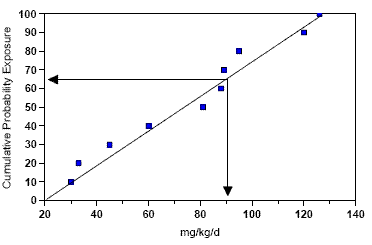Fig. 2
Example of Method 2
(Comparison of Exposure Distribution with Point Estimate for Effects).
The point estimate for effects (90 mg/kg/day) has a 35% probability of being exceeded.

Top of page

## Method 3: Comparison of Exposure and Effects Distributions

Where sufficient data exists to provide meaningful distributions of both exposure and effects, these joint distributions can be compared to determine the extent of overlap. Risk can be expressed as a probability of exceedance of a fixed exposure level. Changes in the magnitude and likelihood of effects can be predicted for different exposure scenarios.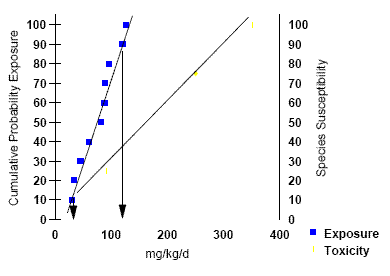Fig. 3a
Example of Method 3
(Comparison of Exposure and Effects Distributions) using Toxicity Data Set 1. The y1 axis represents the % cumulative probability distribution for exposure and the y 2 axis represents the % species sensitivity or mortality.

Top of page

### Examples of Method 3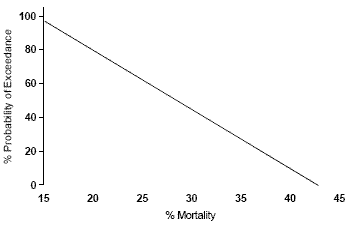Fig. 3b
Example of Method 3
(Comparison of Exposure and Effects Distributions) using Toxicity Data Set 1 where % probability is represented as an exceedance for varying % mortality (or species sensitivity).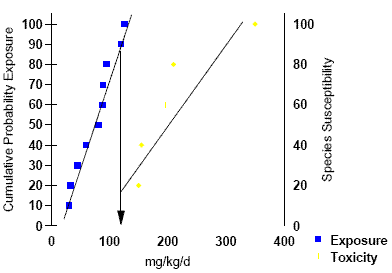Fig. 4
Example of Method 3
(Comparison of Exposure and Effects Distributions) using Toxicity Data Set 2.Fig. 5
Example of Method 3
(Comparison of Exposure and Effects Distributions) using Toxicity Data Set 3.

Top of page

## Method 4: Distribution-Based Quotients

In the Distribution-Based Quotient Method, each individual quotient represents a ratio of exposure to toxicity. The exposure and effects distributions are integrated using Monte Carlo simulations to sample values from distributions of exposure and toxicity to generate a probabilistic distribution of quotients. Risk is expressed from a probability distribution of quotient values, and the probability of the quotient exceeding 1 or any other quotient value. For example " There is a 20% probability that exposure levels exceed effect levels (based on a quotient of 1).Fig. 6
Individual distributions of exposure (graph 1) and toxicity (graphs 2 and 3).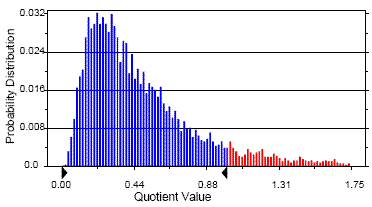Fig. 7a
An example of ADistribution-based Quotients@ (Method 4) based on Toxicity Data Set 1 which contains multiple LD50 values. The right arrow shows a quotient value of 1.0 (equal to the 90th% tile).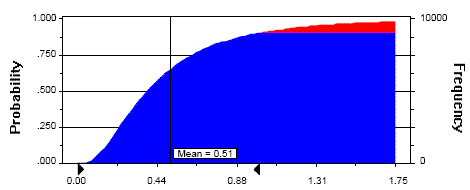Fig. 7b
Distribution-based Quotients@ (Method 4) based on Toxicity Data Set 1 illustrated as a Cumulative Probability plot. The right arrow shows a quotient value of 1.0 (equal to the 90th%tile).

Top of page

## Method 5: Integration of Exposure and Effects Distributions

This method differs from Distribution-Based Quotients (Method 4) in that the quotient is replaced with a mortality response function so that the results of the risk assessment can be expressed as a probability of a certain magnitude of mortality (or some other effect). Three example models were developed. Assumptions of each example Model:

Example Model Assumptions
Parameter Model 1Model 2Model 3
Dose (exposure) (D) Lognormal DistributionLognormal DistributionLognormal Distribution
LD50 Fixed ValueNormal DistributionNormal Distribution
Slope Fixed ValueNormal DistributionNormal Distribution
Lab to Field Extrapolation Uncertainty Factor (UF) noneNoneUF= 75% Probability that the Field LD50 is within 2X Lab LD50
N Individuals 202020
N Simulations 500500500
Tolerance of each Individual (T) T= LD50*10^(z/slope)
z=standard normal distribution (x=0, σ =1)
T= LD50*10^(z/slope)
z=standard normal distribution (x=0, σ =1)
T= (LD50*UF)*10^(z/slope)
z=standard normal distribution (x=0, σ =1)
Fate of Each Individual if D > T then mortality
if D < T then survival
if D > T then mortality
if D < T then survival
if D > T then mortality
if D < T then survival

The probability distributions shift to the right (the probability of mortality increases) as more uncertainty is considered in the model. This results from point estimates being replaced by distributions (Model 2 compared to Model 1) and uncertainty that was previously not explicit being quantified in the model (Model 3 compared to Model 2). The contribution of each source of uncertainty can be explored further in a sensitivity analysis.

Top of page

### The parameter assumptions in Models 1, 2 and 3.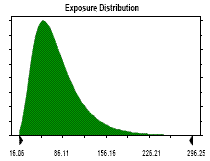Exposure Distribution
Lognormal distribution with parameters:
5% - tile 31.02
95% - tile 153.37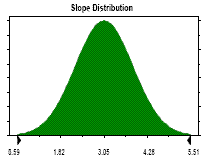Slope Distribution
Normal distribution with parameters:
5% - tile 1.70
95% - tile 4.40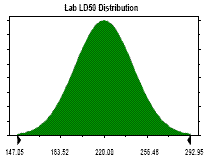Lab LD50 Distribution
Normal distribution with parameters:
5% - tile 180.00
95% - tile 260.00Model 1 Results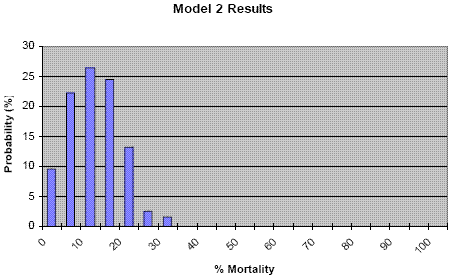Model 2 Results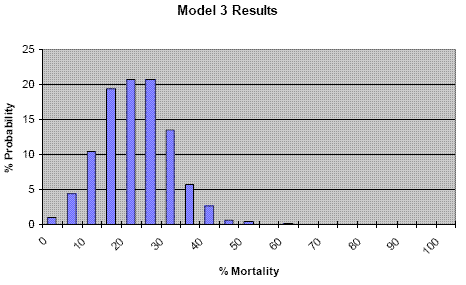Model 3 Results

Top of page

## Levels of Refinement

The "Level of Refinement" refers to the extent that biological realism, risk and uncertainty are incorporated in the risk characterization and how well actual risk is described. In general the progression from lower to higher levels of refinement is based on:

• Point estimates for parameters in the exposure assessment are replaced with distributions

• Additional parameters in the exposure model are considered.

• Increased spatial realism. Both treated and untreated habitats are considered

• An improved estimate of mg/kg/b.w. per unit time for test animals

• Number of species tested is increased

• Pattern of exposure in toxicity test is refined

• More uncertainty is explicitly considered in the analysis

• Decreased uncertainty in the estimate of actual risk

Resource requirements will increase with level of refinement due to the development of additional data to support the probabilistic risk assessment and increased dependence on more complex models. Essentially the data allows parameters or factors used in the exposure or effects analysis that are unaccounted for in earlier levels (e.g., defaulted to 1) to be become explicit at higher levels of refinement. Parameters that are represented by point estimates may incorporate Probability Distribution Functions (PDFs) at higher levels of refinement. This concept is represented in Figure 11.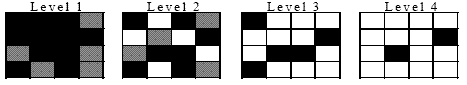Figure 11
Parameter representation in analysis at different Levels of Refinement

##### Legend
•= parameter "off" or default to 1
•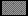= parameter "on", represented by a point
•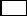= parameter "on", represented by a PDF

Level 1 Level 2 Level 3 Sevel 4 Treated FieldPT=1 Treated Field & Nontarget areasPT < 1 Treated Field, Non-target areas & Drift ZonePT < 1 Landscapeclumpingexplicit sizes pesticide market acute/ gorging = minutes, hoursdietary = hours, daysrepro. = days acute/ gorging = minutes, hoursdietary = hours, daysrepro. = days acute/ gorging = minutes, hoursdietary = hours, daysrepro. = days acute/ gorging = minutes, hoursdietary = hours, daysrepro. = days generic genericfocal focal focal label maximum label maximum label maximumtypical label maximumtypical generic linked to focal speciesgeneric linked to focal speciesgeneric linked to focal speciesindividual cropindividual region acute/gorging mg/kg max (no set time unit)non-gorge: peak daily dose & TWA (mg/kg/d) distribution of gorge dosefrequency of gorgingnon-gorge:daily mg/kg/hr (peak) distributions & distribution of TWA improved distributions (more data)consideration of drift zones (non-gorge)distributions replacing fixed defaults for parametersmore explicit mechanisms improved distributions (more data)field data on focal speciesconsideration of landscape factors in spatially explicit models Acute: 1 LD50 dose-response * UFDietary: 2 LD50 * UFReproduction: 2 NOELS Acute: 2-3 LD50 * UFDietary: 2 LD50 * UF, individual cagingReproduction: 2 NOELS Acute: 4+ LD50 * UFDietary: 2 LD50 * UF, individual caging, vary exposureReproduction: 2 NOELS, vary exposure, aviary study Field options but only in combination with exposure assessments Deterministic Quotients Acute: methods 2-5 as appropriateDietary: methods 2-5 as appropriateRepro.: methods 2 Acute: methods 2-5 as appropriateDietary: methods 2-5 as appropriateRepro.: methods 2 Acute: methods 2-5 as appropriateDietary: methods 2-5 as appropriateRepro.: methods 2 Quotient Probability distribution specific to method selected Probability distribution specific to method selected Probability distribution specific to method selected

Top of page

US Environmental Protection Agency, 1992. Framework for Ecological Risk Assessment. US Environmental Protection Agency, EPA/630/R-92/001.

US Environmental Protection Agency, 1998. Guidelines for Ecological Risk Assessment. US Environmental Protection Agency, EPA/630/R-95/002Fa.

Top of page